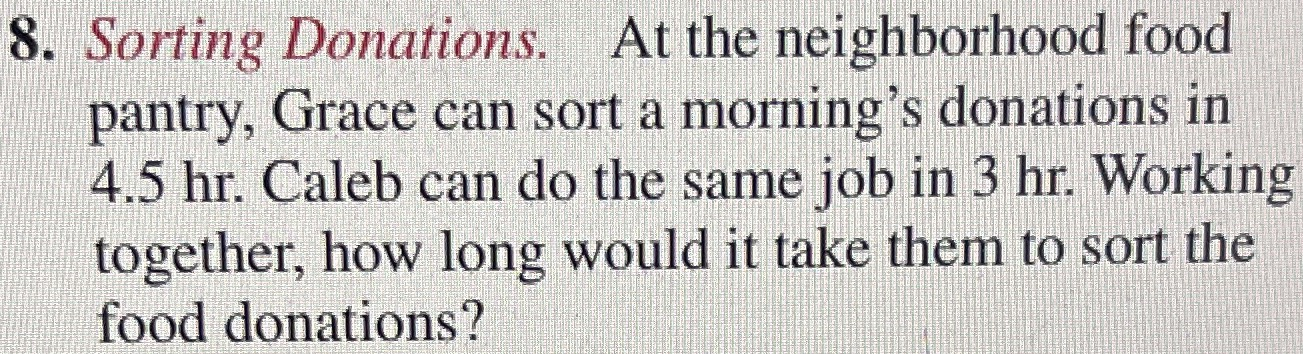### Still have math questions?

Arithmetic
Question8. Sorting Donations. At the neighborhood food pantry, Grace can sort a morning's donations in

$$4.5 hr$$ . Caleb can do the same job in $$3 hr$$ . Working together, how long would it take them to sort the food donations?

1÷($$\frac{1}{3}$$+$$\frac{1}{4.5}$$)=1.8 hr